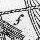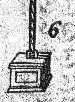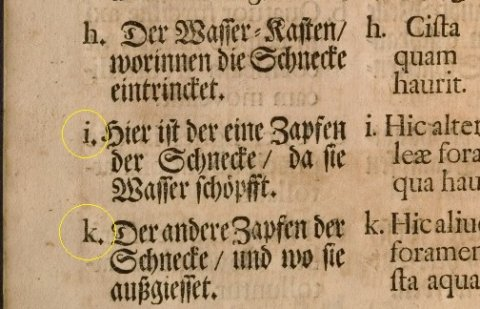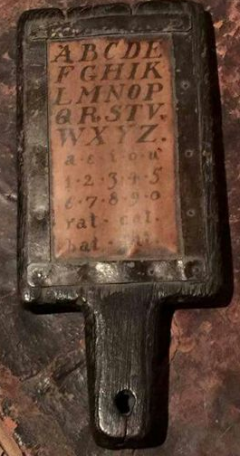Cracking codes / Alphabetic values

# Alphabetic values

 Letters can and have numeric values. this is clearly shown in Besslers first and second drawing of the Kassel wheel.  The first have letters as parts list, where the second has numbers. Clearly making letters and numbers equivalent for numbering the parts.Bessler used a modified version of this equivalent/substiture method. The special substitute scheme makes up one part of "the Orffyrean Code"    In general we can say that the following alphabet scheme, using 24 individual letters was still the leading alphabet/substitute table: (Where U=V and I=J)   An example can be seen here in one of Orfferus' parts lists. Since "I" and "J" is the same letter, the letter "J" is omitted:The alphabet values:   A  = 1 B  = 2C  = 3 D  = 4E  = 5F  = 6 G  = 7 H  = 8I/J = 9 K = 10L =  11 M = 12N = 13 O = 14P = 15Q = 16R = 17S =  18T =  19U/V = 20 W = 21X = 22Y = 23Z = 2424 Letter alphabet used in schools.